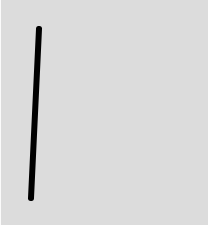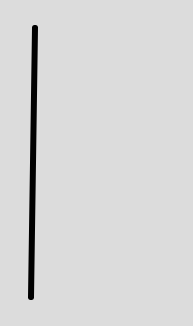Related Articles

# p5.js | line() Function

• Last Updated : 10 Apr, 2019

The line() function is an inbuilt function in p5.js which is used to draw a line. In order to change the color of the line stroke() function is used and in order to change the width of the line strokeWeight() function is used.

Syntax:

`line(x1, y1, x2, y2)`

or

`line(x1, y1, z1, x2, y2, z2)`

Parameters: This function accepts six parameters as mentioned above and described below:

• x1: This parameter takes the x-coordinate of the first point.
• y1: This parameter takes the y-coordinate of the first point.
• z1: This parameter takes the z-coordinate of the first point.
• x2: This parameter takes the x-coordinate of the second point.
• y2: This parameter takes the y-coordinate of the second point.
• z2: This parameter takes the z-coordinate of the second point.

Below programs illustrates the line() function in P5.js:

Example 1: This example uses line() function to draw a line without using z-coordinate.

 `function` `setup() {`` ` `    ``// Set the canvas size ``    ``createCanvas(400, 400);``}``  ` `function` `draw() {``     ` `    ``// Set the background color``    ``background(220);``     ` `    ``// Set the stroke weight``    ``strokeWeight(6);``     ` `    ``//x1, y1 = 38, 31; x2, y2 = 300, 20;``    ``// Use line() function to draw line``    ``line(38, 31, 30, 200); ``}`

Output:Example 2: This example uses line() function to draw the line using z-coordinate.

 `function` `setup() {`` ` `    ``// Set the canvas size ``    ``createCanvas(400, 400);``}``  ` `function` `draw() {``     ` `    ``// Set the background color``    ``background(220);``     ` `    ``// Set the stroke weight``    ``strokeWeight(6);``     ` `    ``//x1, y1, z1 = 38, 31, 34; ``    ``// x2, y2, z2 = 300, 200, 45;``    ``// Use line() function to draw line``    ``line(38, 31, 34, 300, 200, 45); ``}`

Output:Reference: https://p5js.org/reference/#/p5/line

My Personal Notes arrow_drop_up